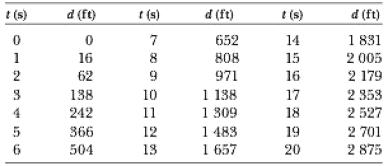# Members of a skydiving club were given the following data to use in planning their jumps. In the table, d is the distance fallen from rest by a skydiver in a “free-fall stable spread position” versus the time of fall t . (a) Convert the distances in feet into meters, (b) Graph d (in meters) versus t . (c) Determine the value of the terminal speed v t by finding the slope of the straight portion of the curve. Use a least-squares fit to determine this slope.### Physics for Scientists and Enginee...

9th Edition
Raymond A. Serway + 1 other
Publisher: Cengage Learning
ISBN: 9781305116399

#### Solutions

Chapter
Section### Physics for Scientists and Enginee...

9th Edition
Raymond A. Serway + 1 other
Publisher: Cengage Learning
ISBN: 9781305116399
Chapter 6, Problem 6.60AP
Textbook Problem
57 views

## Members of a skydiving club were given the following data to use in planning their jumps. In the table, d is the distance fallen from rest by a skydiver in a “free-fall stable spread position” versus the time of fall t. (a) Convert the distances in feet into meters, (b) Graph d (in meters) versus t. (c) Determine the value of the terminal speed vt by finding the slope of the straight portion of the curve. Use a least-squares fit to determine this slope.(a)

To determine

The distances in terms of meters.

### Explanation of Solution

The Conversion of feet into meters

1ft=0.3048m

Convert the distances in feet to meters.

 t(s) d(ft) d(m) 0 0 0 1 16 4.88 2 62 18.9 3 138 42.1 4 242 73

(b)

To determine

The position versus time graph.

(c)

To determine

The terminal speed using the slope of the straight portion of the curve.

### Still sussing out bartleby?

Check out a sample textbook solution.

See a sample solution

#### The Solution to Your Study Problems

Bartleby provides explanations to thousands of textbook problems written by our experts, many with advanced degrees!

Get Started

Find more solutions based on key concepts
Name the following molecular compounds a ClF4 b CS2 c PF3 d SF6

General Chemistry - Standalone book (MindTap Course List)

In adults with vitamin D deficiency, poor bone mineralization can lead to pellagra. pernicious anemia. scurvy. ...

Nutrition: Concepts and Controversies - Standalone book (MindTap Course List)

Which weighs more, 0.001 g of water or 1 mg of water?

Introductory Chemistry: A Foundation

What is blood plasma, and what is its function?

Human Biology (MindTap Course List)

What is the nucleolus, and what is its function?

Biology: The Dynamic Science (MindTap Course List)

Define the following terms: a. chromosome b. chromatin

Human Heredity: Principles and Issues (MindTap Course List)